Maps each level to an evenly spaced hue on the colour wheel. It does not generate colour-blind safe palettes.

## Usage

scale_colour_hue(
...,
h = c(0, 360) + 15,
c = 100,
l = 65,
h.start = 0,
direction = 1,
na.value = "grey50",
aesthetics = "colour"
)

scale_fill_hue(
...,
h = c(0, 360) + 15,
c = 100,
l = 65,
h.start = 0,
direction = 1,
na.value = "grey50",
aesthetics = "fill"
)

## Arguments

...

Arguments passed on to discrete_scale

palette

A palette function that when called with a single integer argument (the number of levels in the scale) returns the values that they should take (e.g., scales::hue_pal()).

breaks

One of:

• NULL for no breaks

• waiver() for the default breaks (the scale limits)

• A character vector of breaks

• A function that takes the limits as input and returns breaks as output. Also accepts rlang lambda function notation.

limits

One of:

• NULL to use the default scale values

• A character vector that defines possible values of the scale and their order

• A function that accepts the existing (automatic) values and returns new ones. Also accepts rlang lambda function notation.

drop

Should unused factor levels be omitted from the scale? The default, TRUE, uses the levels that appear in the data; FALSE uses all the levels in the factor.

na.translate

Unlike continuous scales, discrete scales can easily show missing values, and do so by default. If you want to remove missing values from a discrete scale, specify na.translate = FALSE.

scale_name

The name of the scale that should be used for error messages associated with this scale.

name

The name of the scale. Used as the axis or legend title. If waiver(), the default, the name of the scale is taken from the first mapping used for that aesthetic. If NULL, the legend title will be omitted.

labels

One of:

• NULL for no labels

• waiver() for the default labels computed by the transformation object

• A character vector giving labels (must be same length as breaks)

• An expression vector (must be the same length as breaks). See ?plotmath for details.

• A function that takes the breaks as input and returns labels as output. Also accepts rlang lambda function notation.

guide

A function used to create a guide or its name. See guides() for more information.

expand

For position scales, a vector of range expansion constants used to add some padding around the data to ensure that they are placed some distance away from the axes. Use the convenience function expansion() to generate the values for the expand argument. The defaults are to expand the scale by 5% on each side for continuous variables, and by 0.6 units on each side for discrete variables.

position

For position scales, The position of the axis. left or right for y axes, top or bottom for x axes.

super

The super class to use for the constructed scale

h

range of hues to use, in [0, 360]

c

chroma (intensity of colour), maximum value varies depending on combination of hue and luminance.

l

luminance (lightness), in [0, 100]

h.start

hue to start at

direction

direction to travel around the colour wheel, 1 = clockwise, -1 = counter-clockwise

na.value

Colour to use for missing values

aesthetics

Character string or vector of character strings listing the name(s) of the aesthetic(s) that this scale works with. This can be useful, for example, to apply colour settings to the colour and fill aesthetics at the same time, via aesthetics = c("colour", "fill").

Other colour scales: scale_alpha(), scale_colour_brewer(), scale_colour_continuous(), scale_colour_gradient(), scale_colour_grey(), scale_colour_steps(), scale_colour_viridis_d()

## Examples

# \donttest{
set.seed(596)
dsamp <- diamonds[sample(nrow(diamonds), 1000), ]
(d <- ggplot(dsamp, aes(carat, price)) + geom_point(aes(colour = clarity)))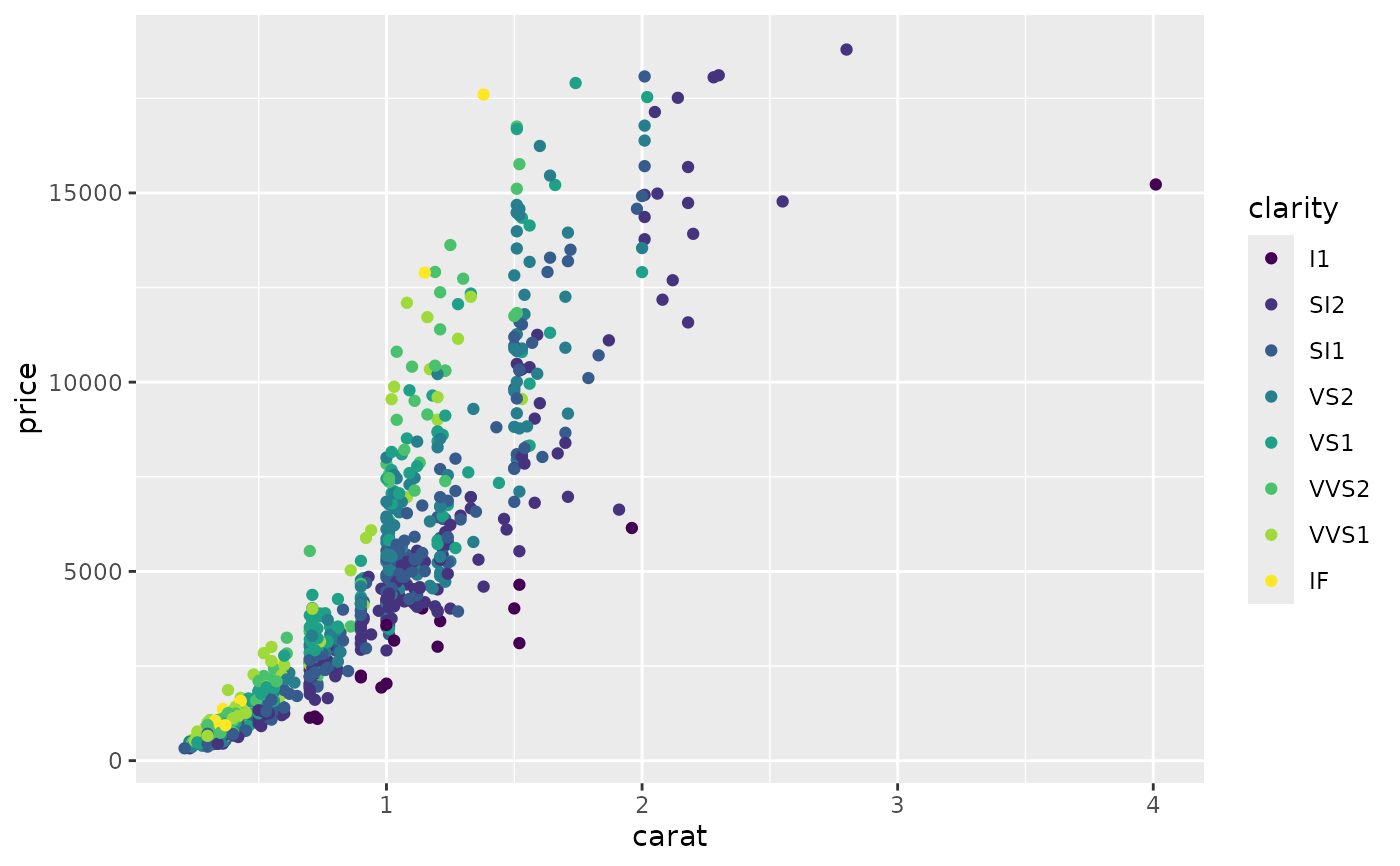# Change scale label
d + scale_colour_hue()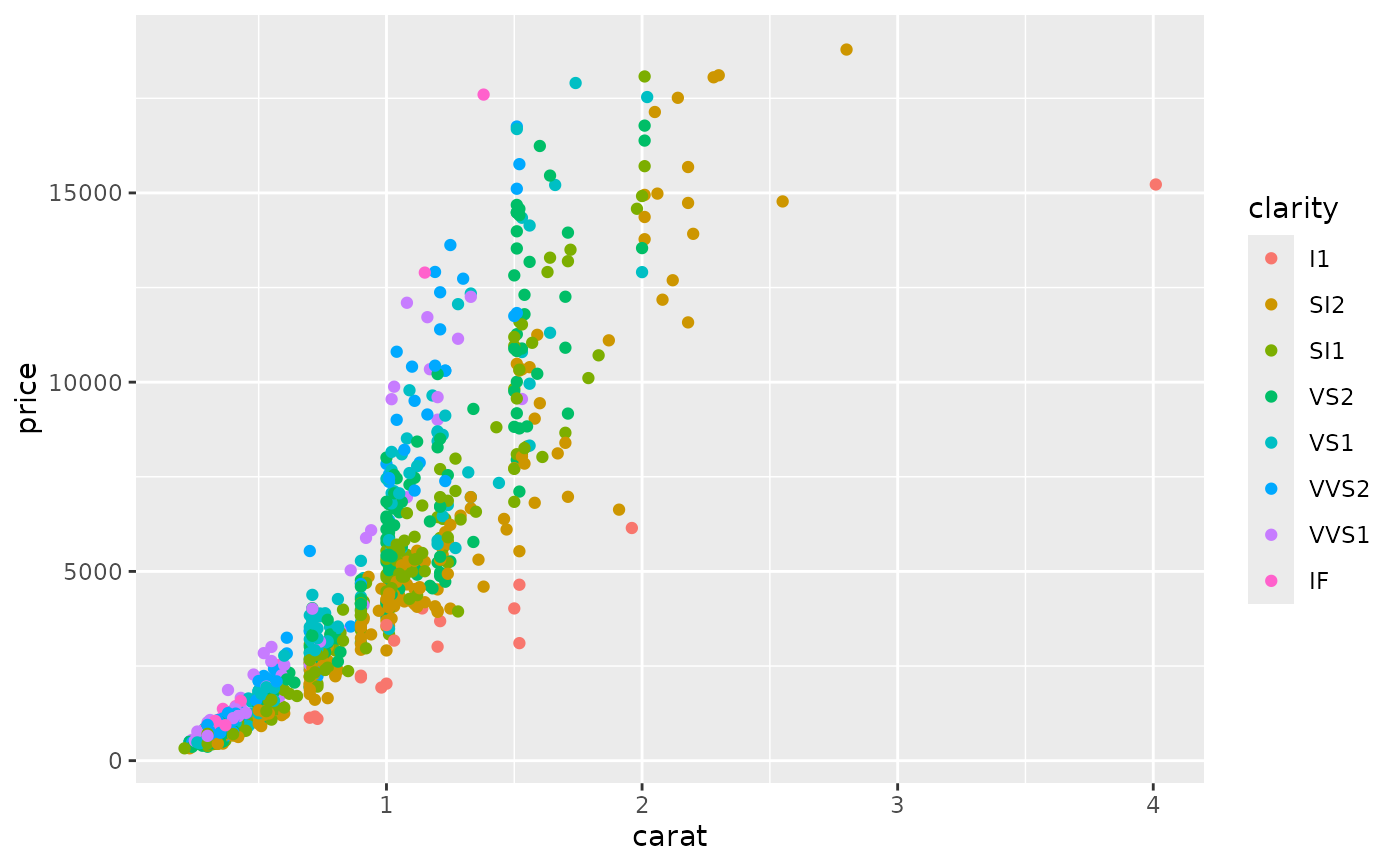d + scale_colour_hue("clarity")d + scale_colour_hue(expression(clarity[beta]))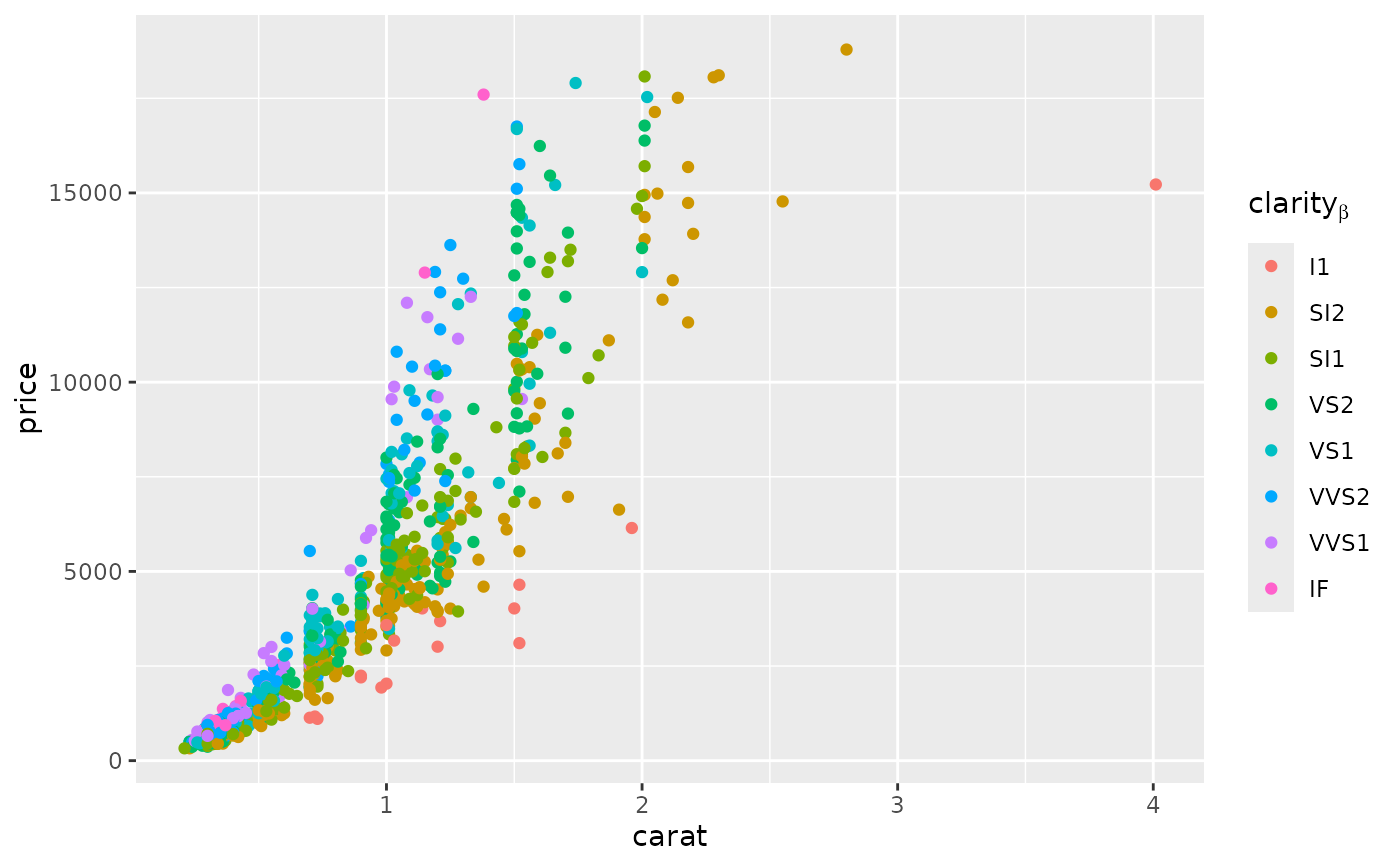d + scale_colour_hue(l = 40, c = 30)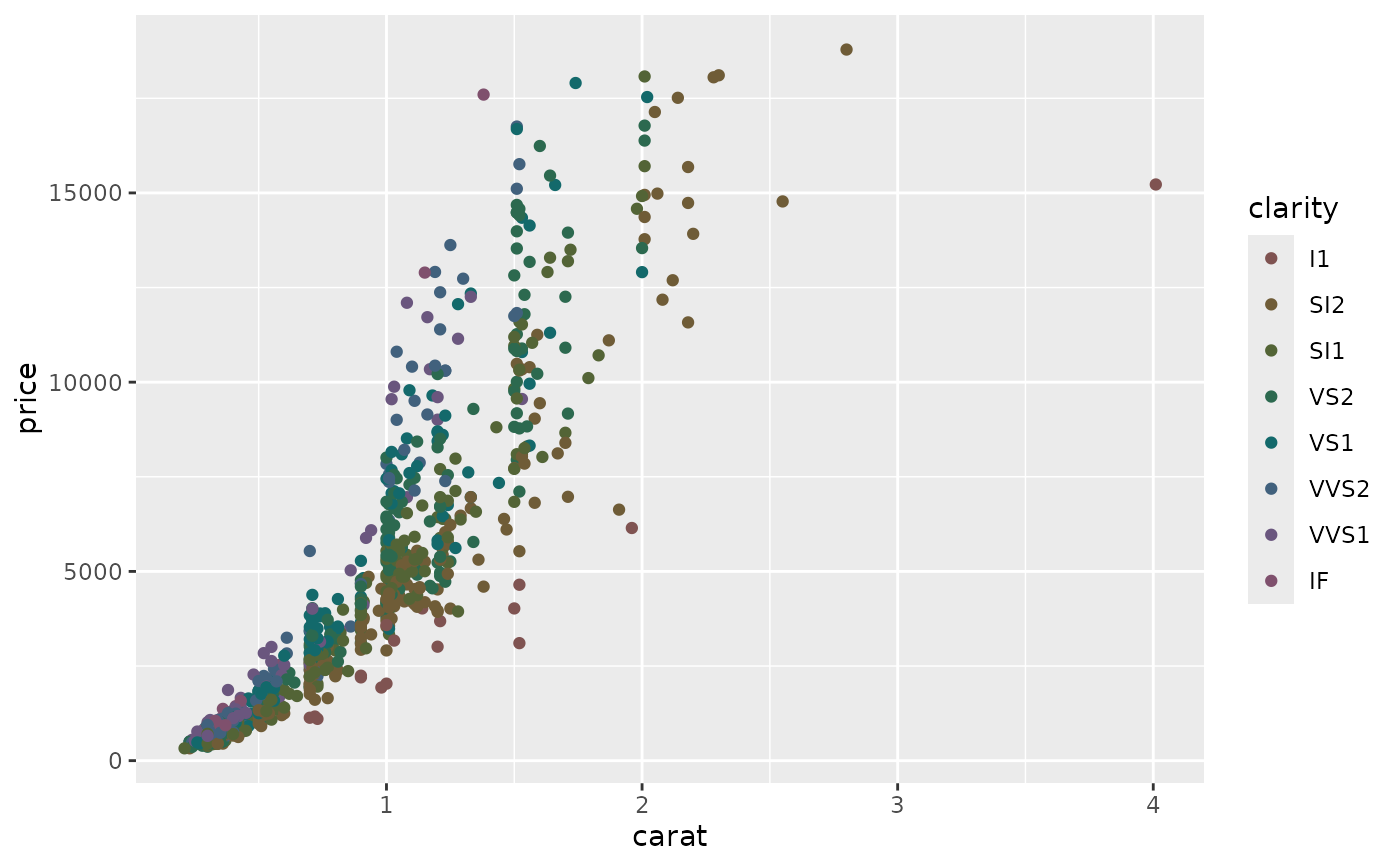d + scale_colour_hue(l = 70, c = 30)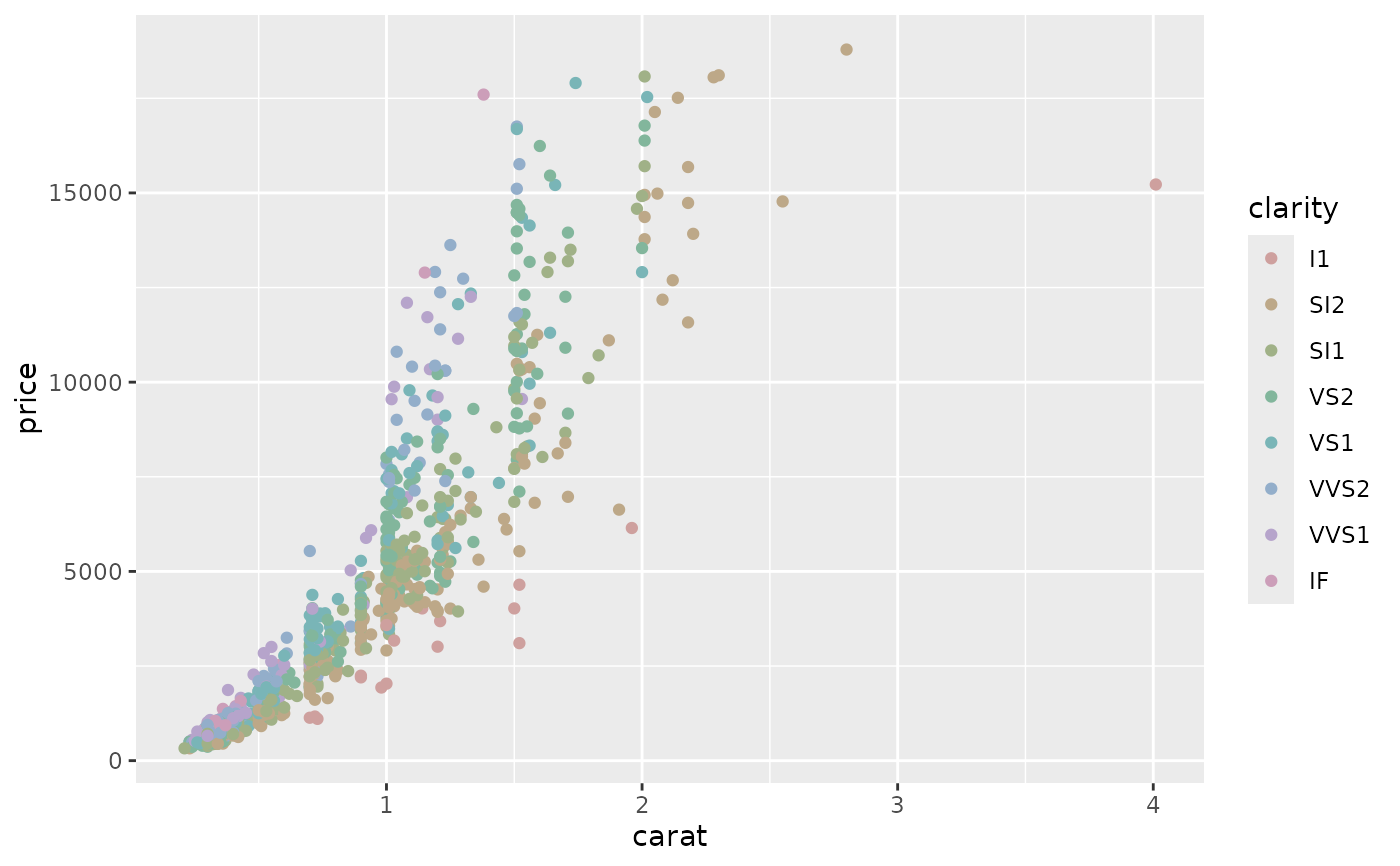d + scale_colour_hue(l = 70, c = 150)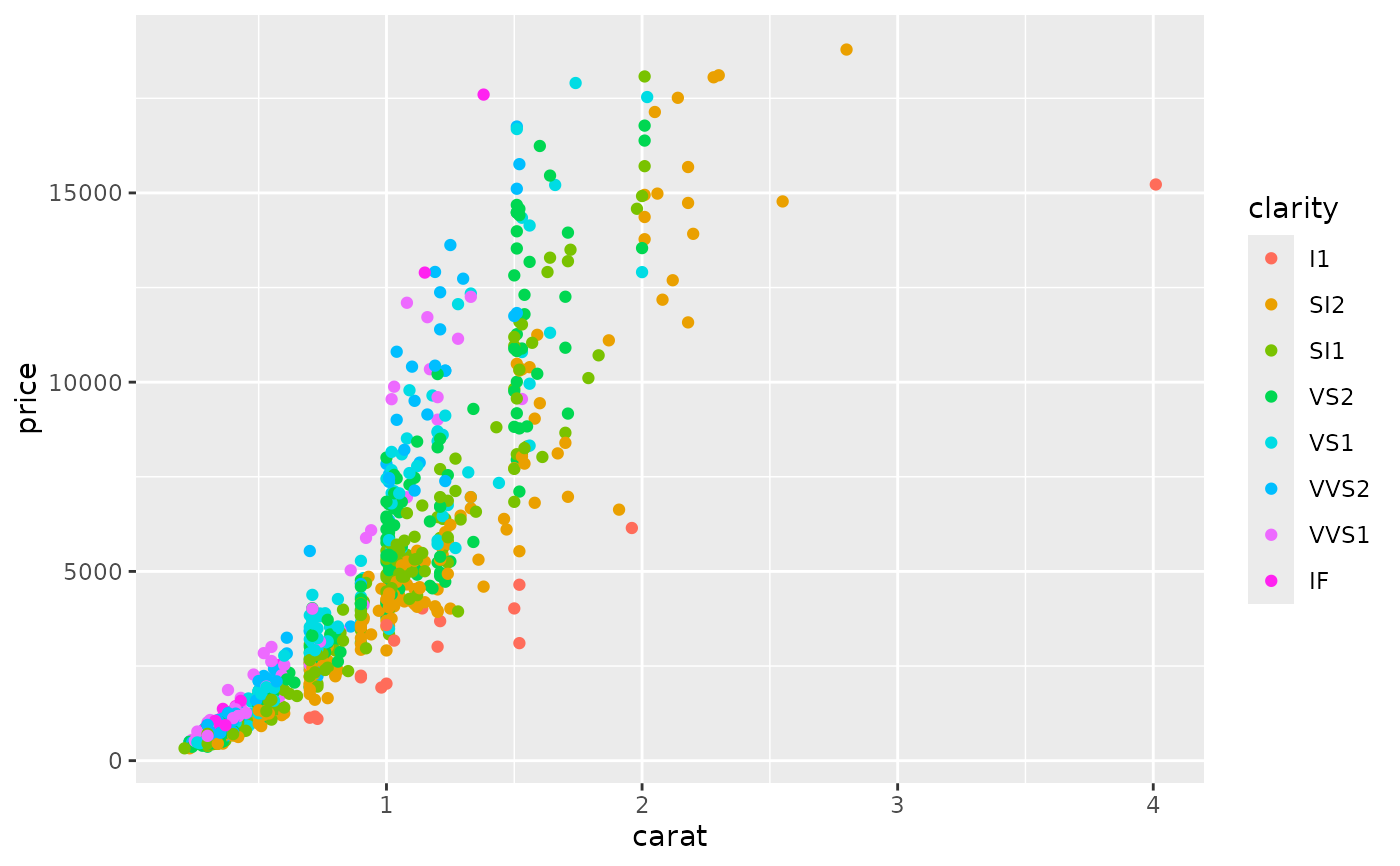d + scale_colour_hue(l = 80, c = 150)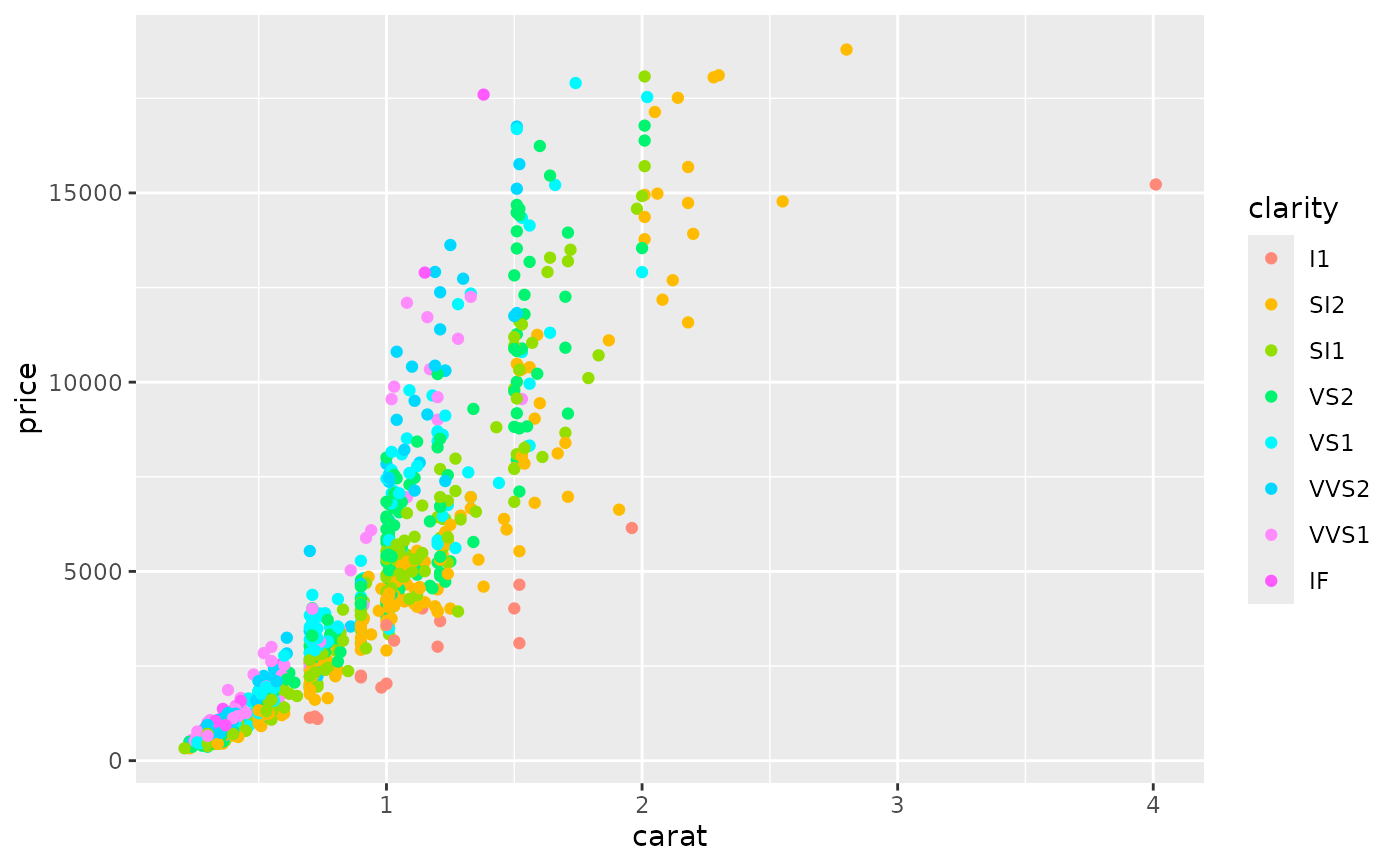# Change range of hues used
d + scale_colour_hue(h = c(0, 90))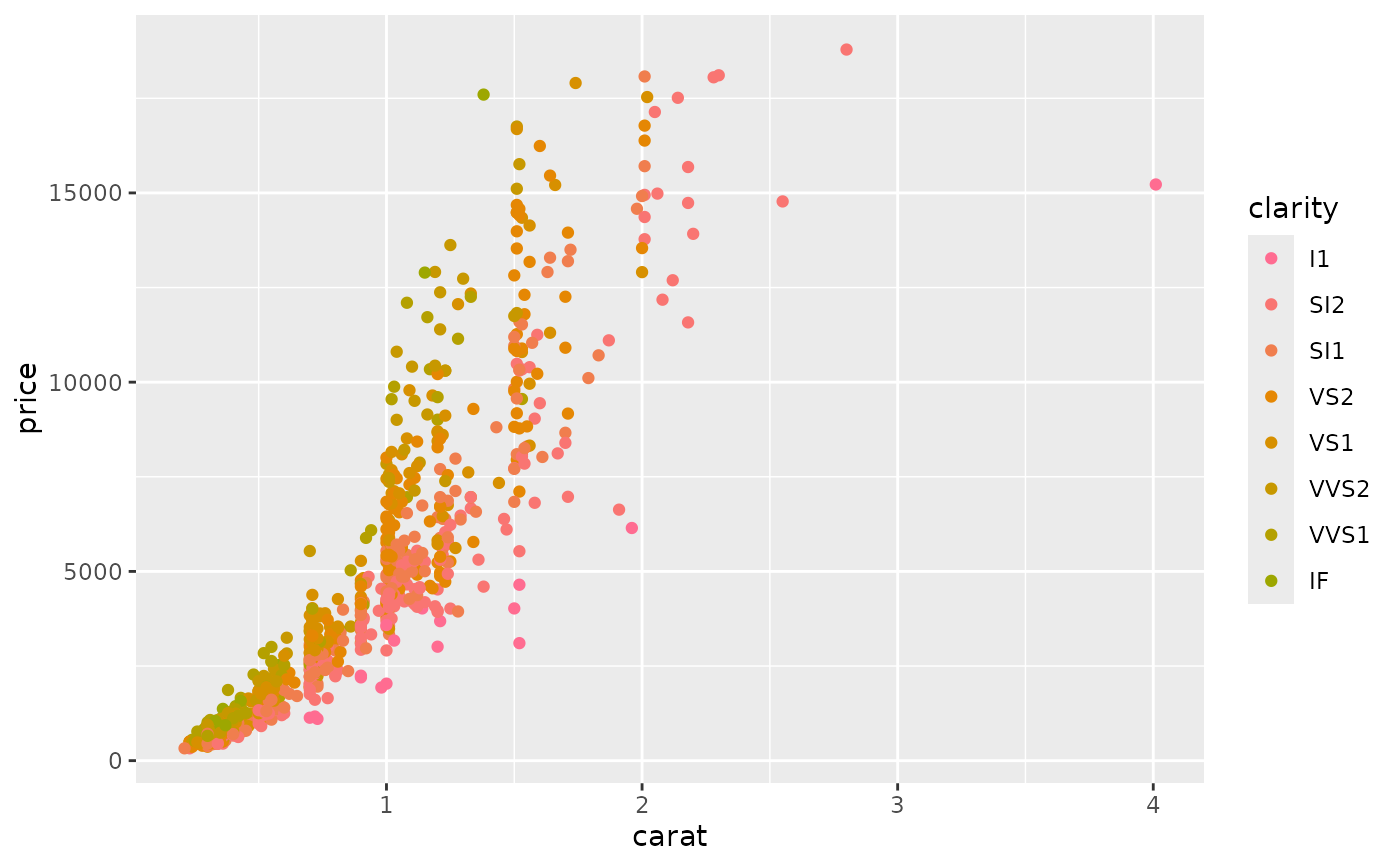d + scale_colour_hue(h = c(90, 180))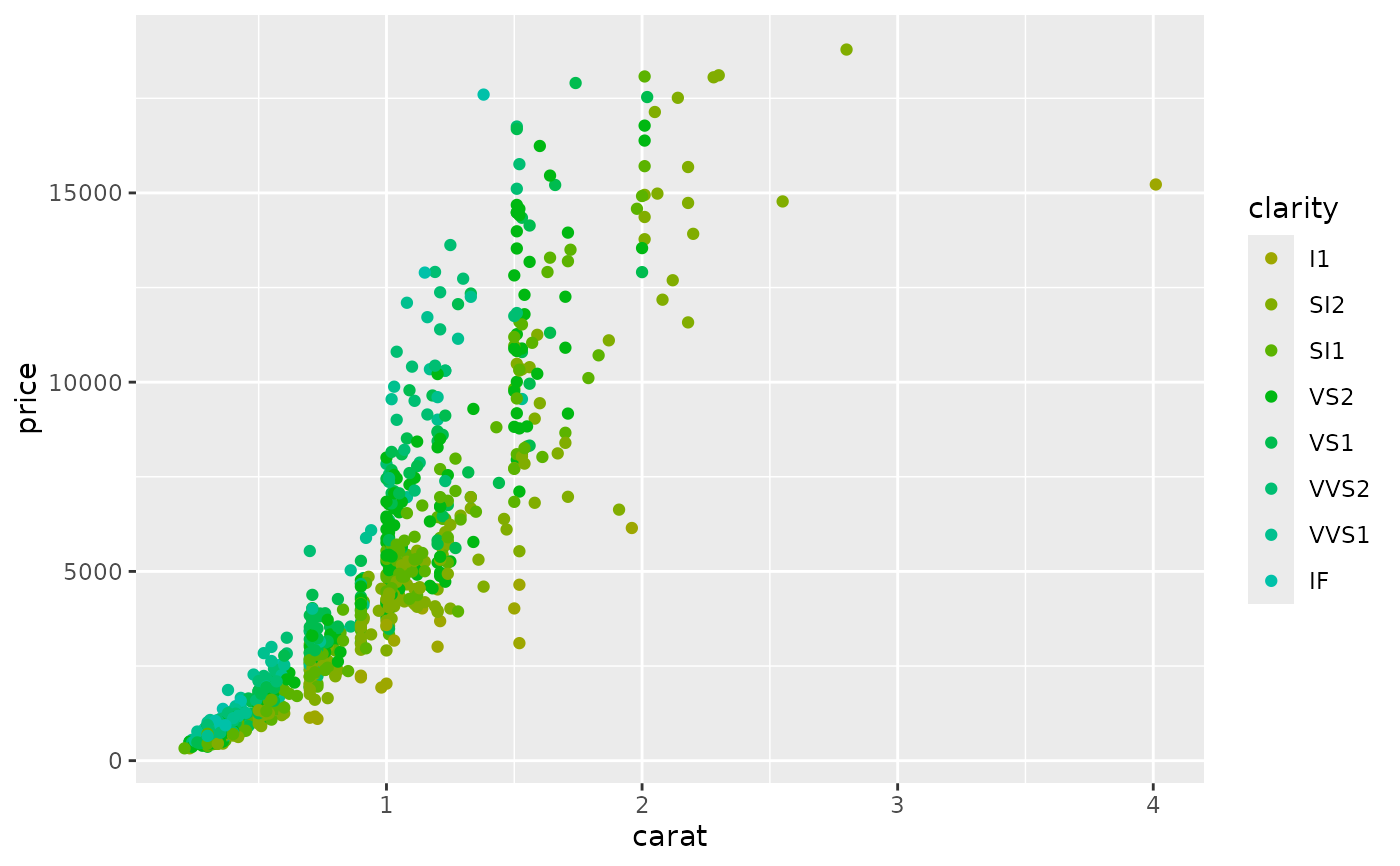d + scale_colour_hue(h = c(180, 270))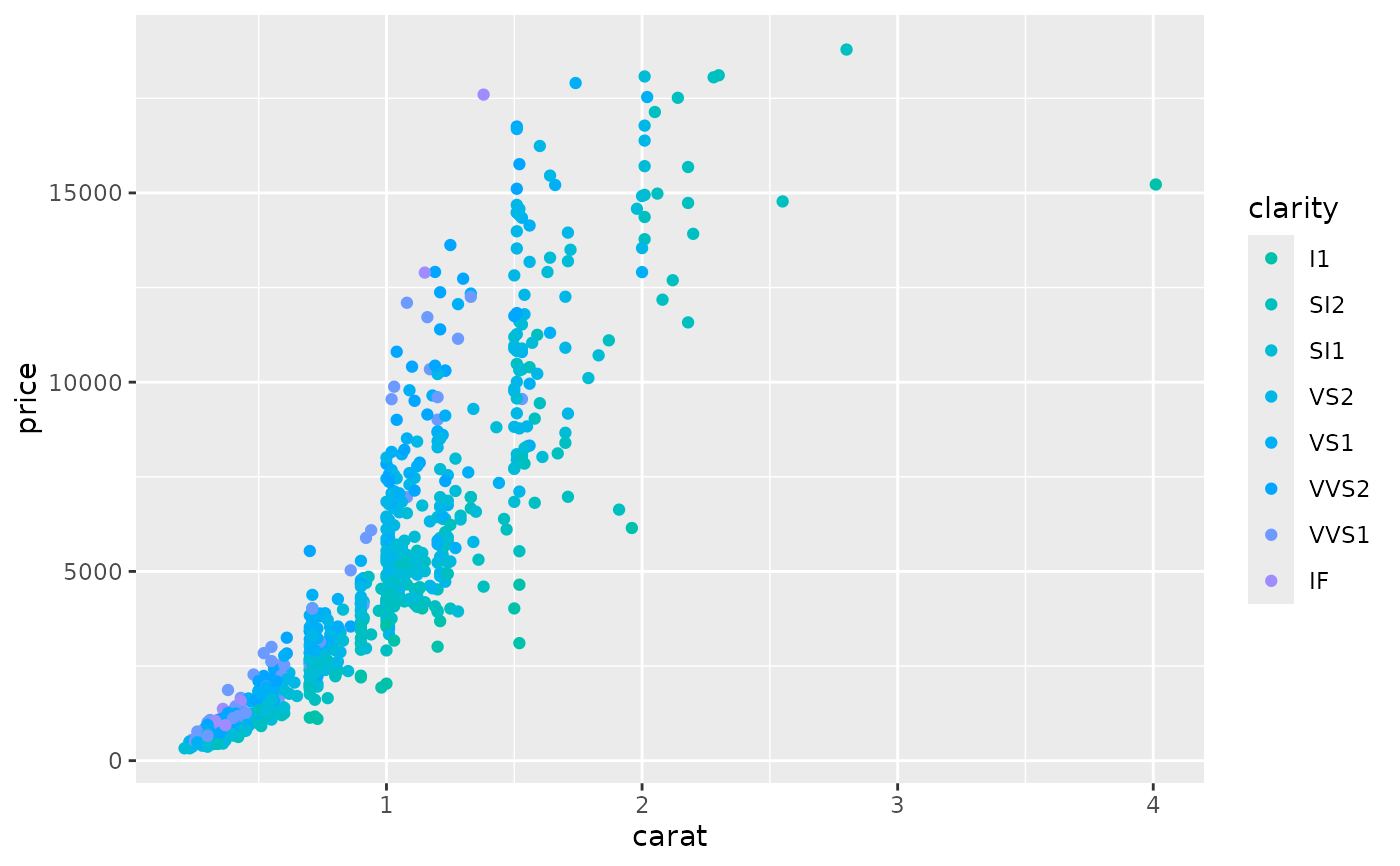d + scale_colour_hue(h = c(270, 360))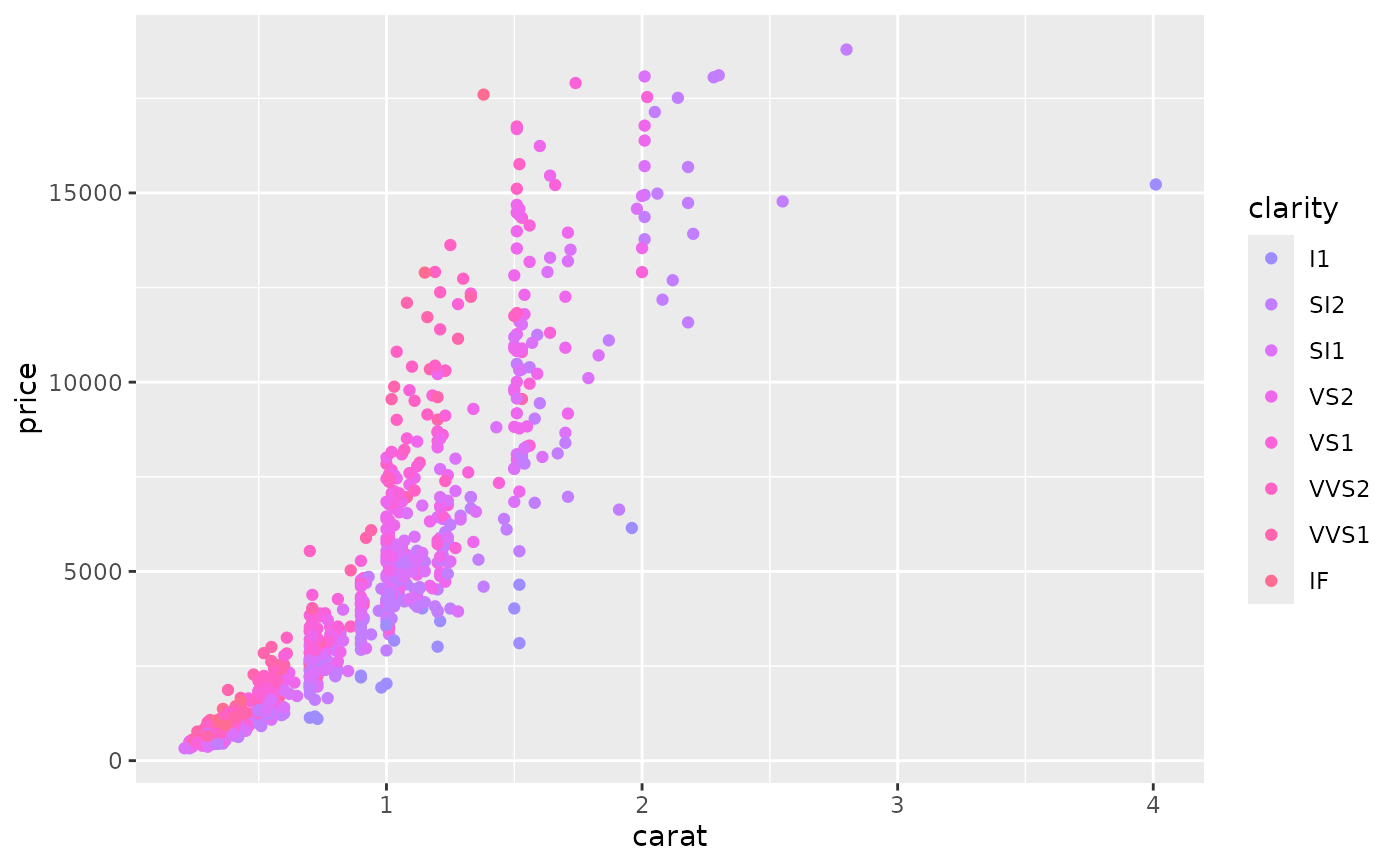# Vary opacity
# (only works with pdf, quartz and cairo devices)
d <- ggplot(dsamp, aes(carat, price, colour = clarity))
d + geom_point(alpha = 0.9)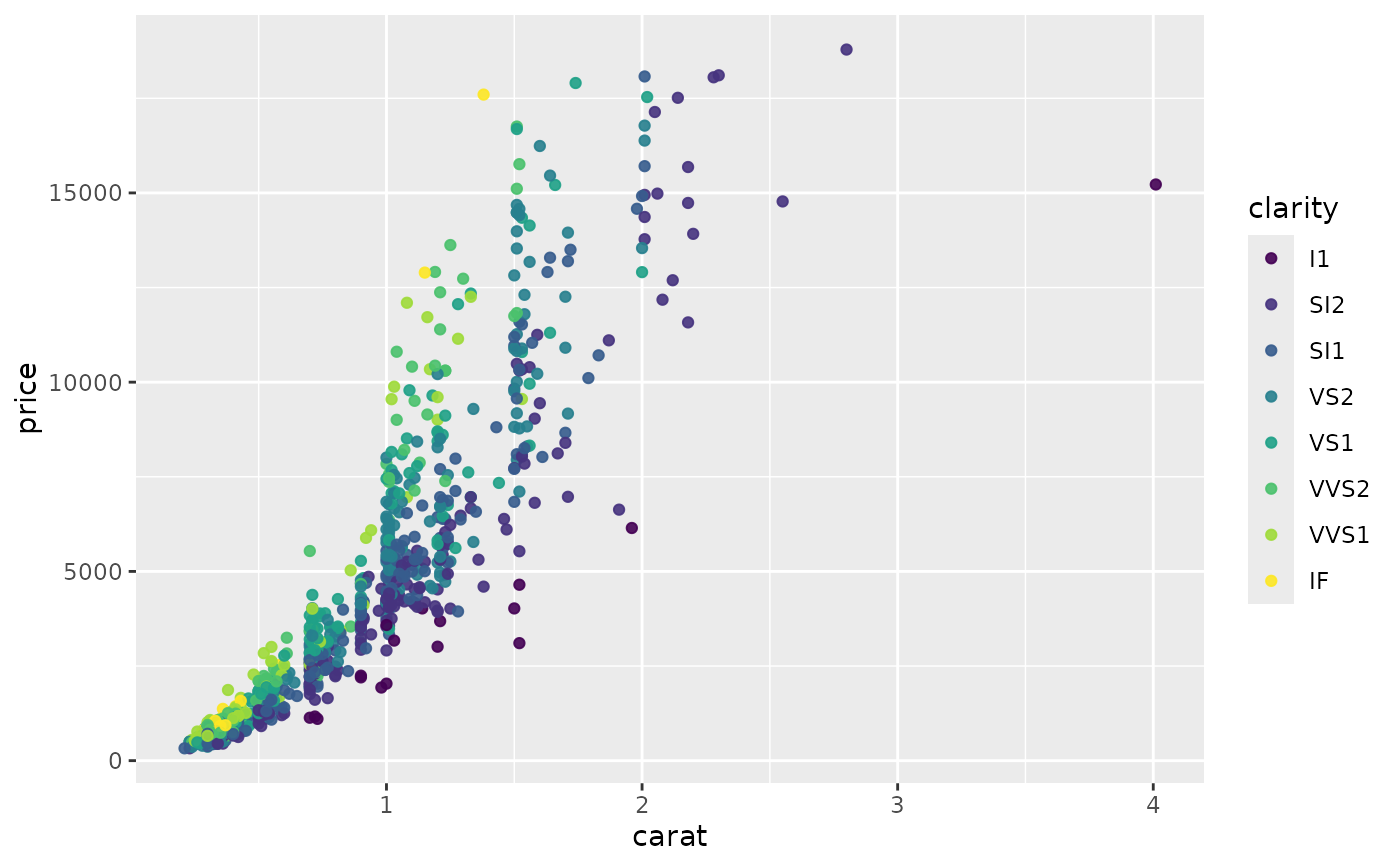d + geom_point(alpha = 0.5)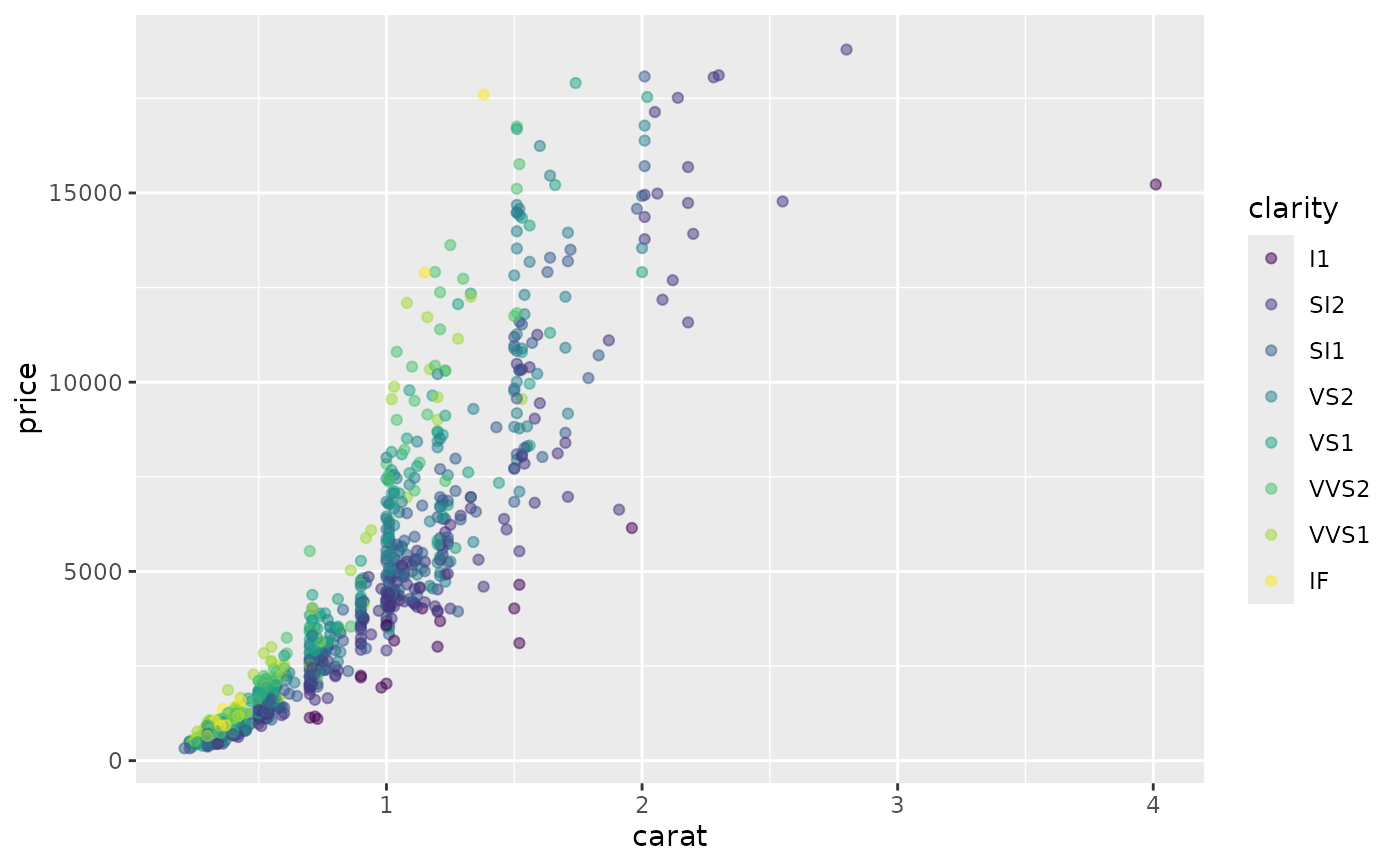d + geom_point(alpha = 0.2)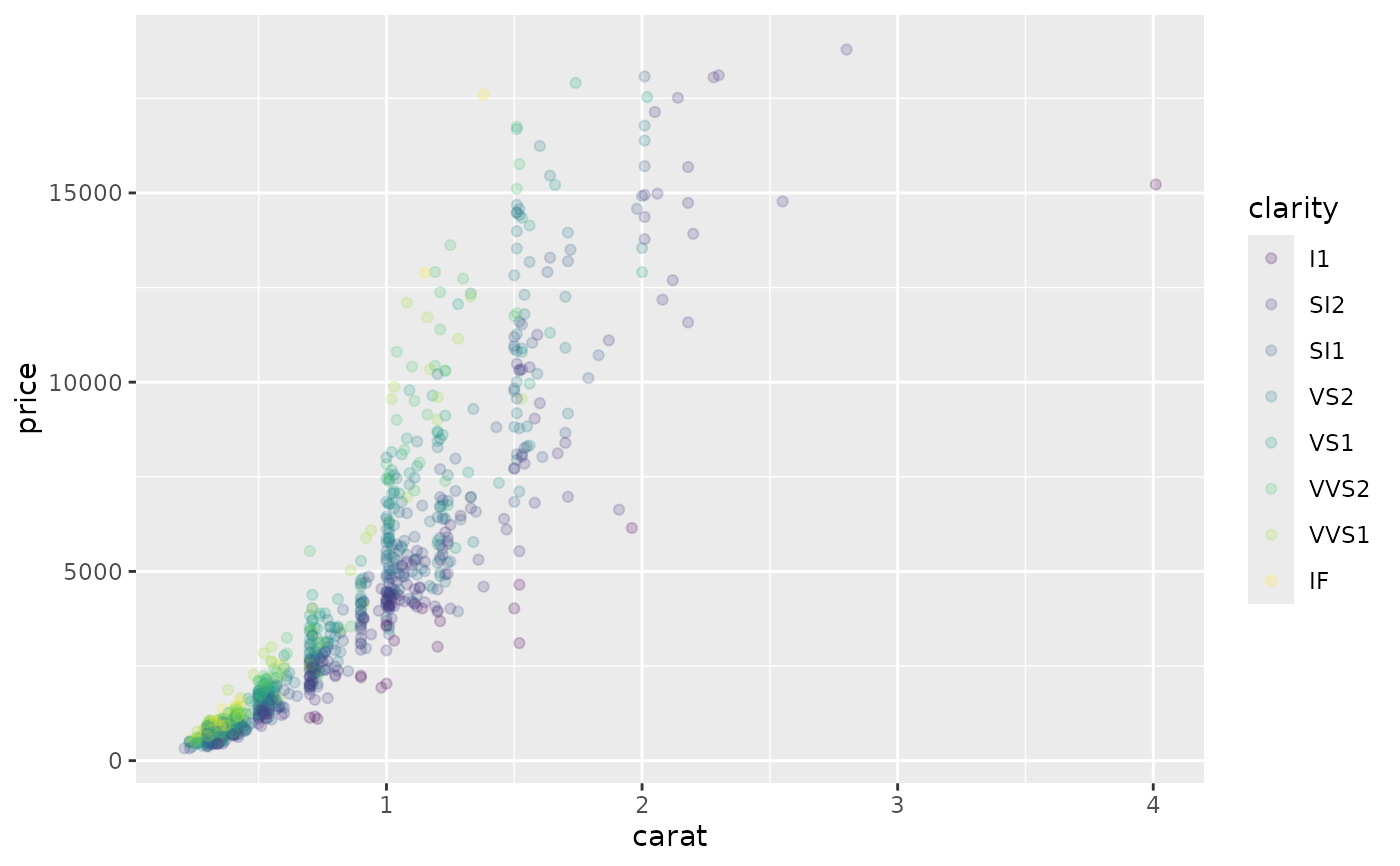# Colour of missing values is controlled with na.value:
miss <- factor(sample(c(NA, 1:5), nrow(mtcars), replace = TRUE))
ggplot(mtcars, aes(mpg, wt)) +
geom_point(aes(colour = miss))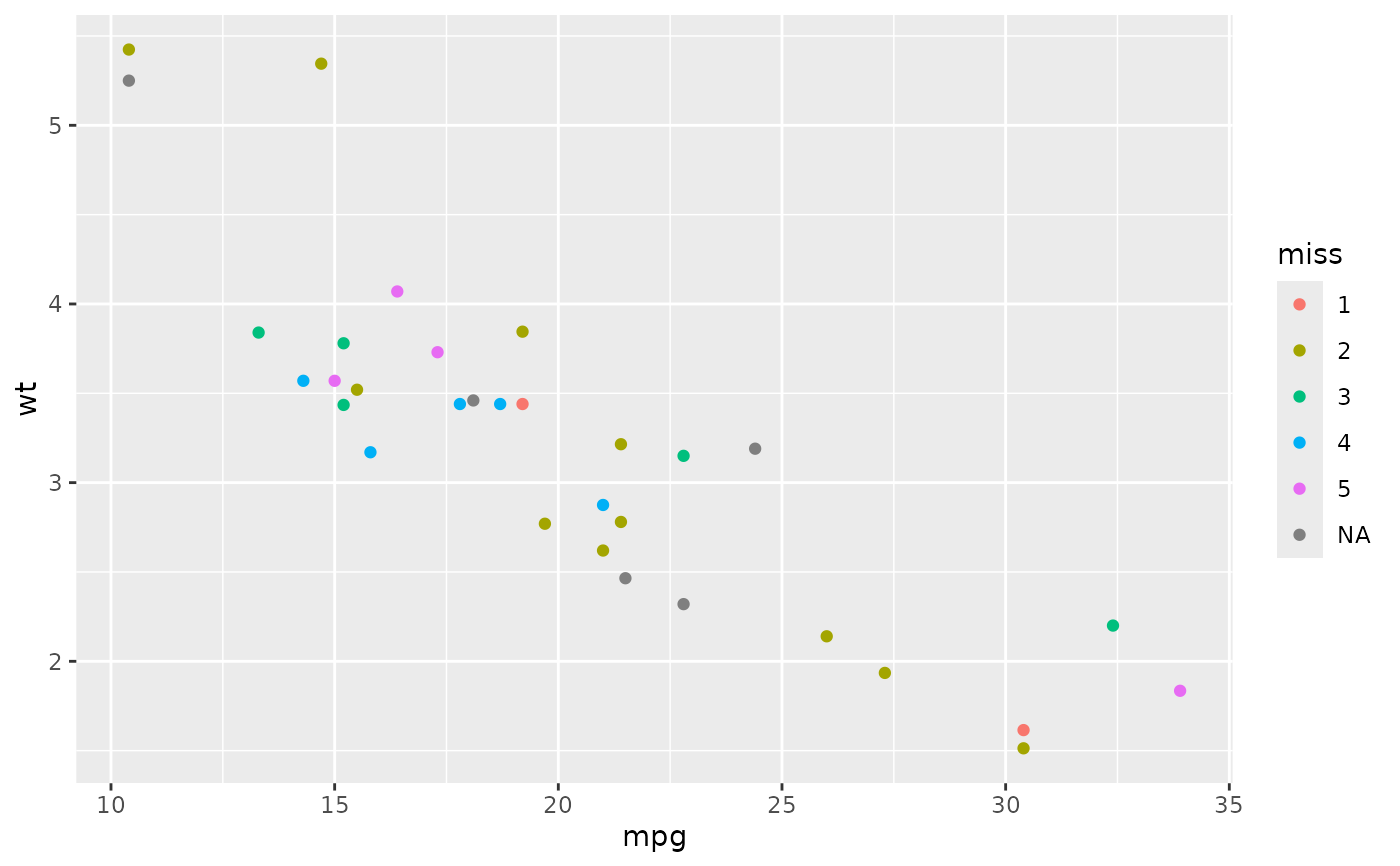ggplot(mtcars, aes(mpg, wt)) +
geom_point(aes(colour = miss)) +
scale_colour_hue(na.value = "black")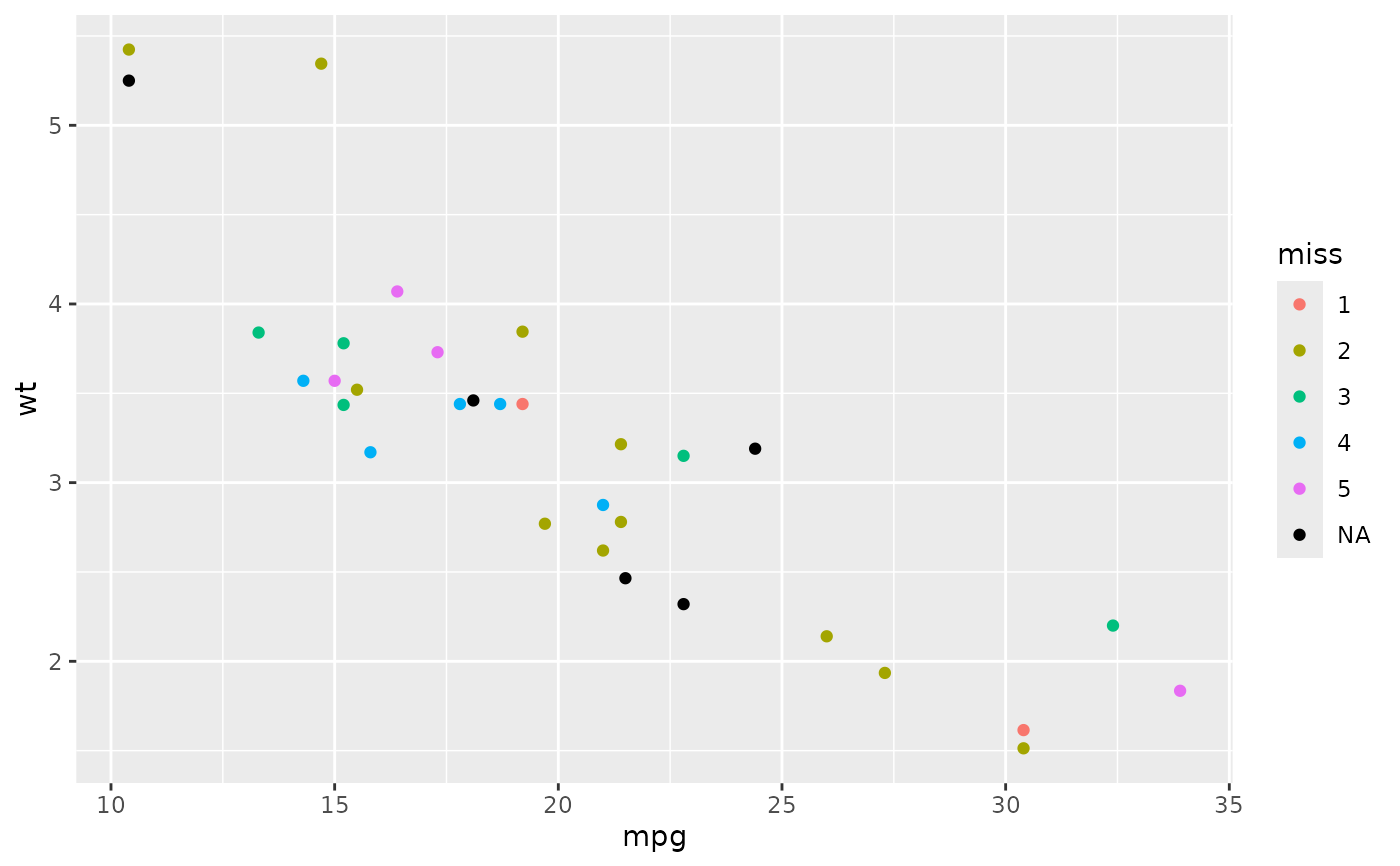# }